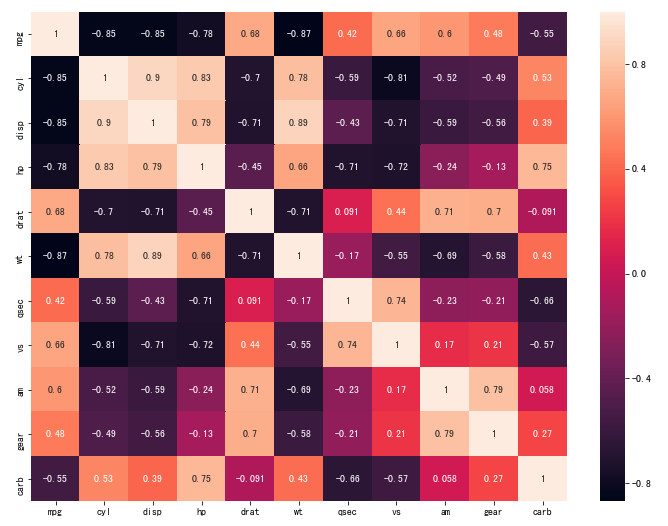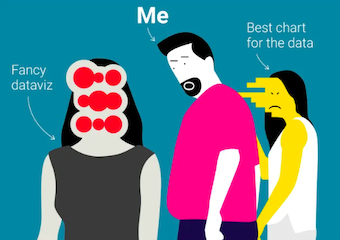DAY 5
0

1. 看劇資料 (CRM資料)
2. 股票資料 (時間序列資料)
3. UCI 資料集

Python的視覺化package主要會使用，seabornMatplotlibplotly等套件，下面視覺化程式。

情境一: 老闆說: 我想知道我們會員資料的Profile概況:

Ex:

ID Gender
Ricky
Lucy

sb.countplot('gender',data=df_sex[['gender']])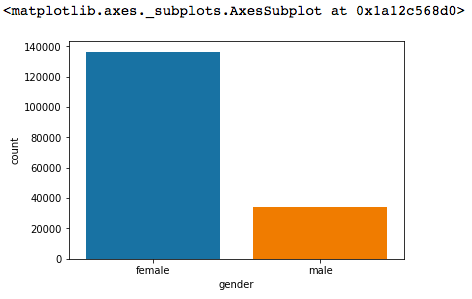age.plot(kind='pie', subplots=True, figsize=(6, 6))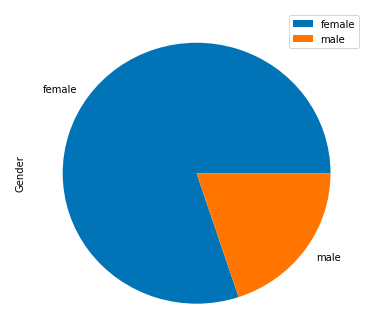sb.distplot(age['age'])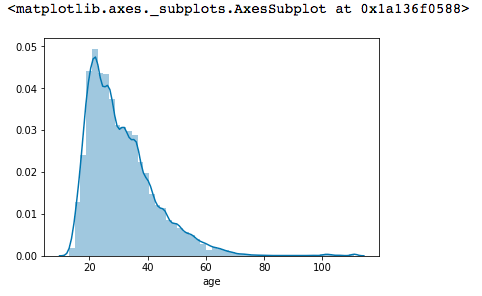squarify.plot(sizes=g['count'], label=g.index, alpha=.8)
plt.axis('off')
plt.show()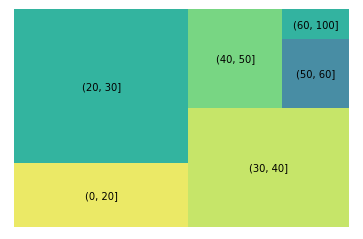情境二: 老闆說: 我想知道我們目前最熱門的連續劇(產品)以及大致的profile狀況

fig, axs = plt.subplots(ncols=3,figsize=(16,8))
sb.barplot(x='count', y=top_drama.index, data=top_drama, ax=axs)
sb.barplot(x='count', y=top_age.index, data=top_age, ax=axs)
sb.barplot(x='count', y=top_sex.index, data=top_sex, ax=axs)情境三: 老闆說: 我想做一些交叉分析，例如：知道劇 (產品) 的使用者年齡分佈

Ex:

f, ax = plt.subplots(figsize=(8, 6))
sb.boxplot(x='event3', y="age", data=data)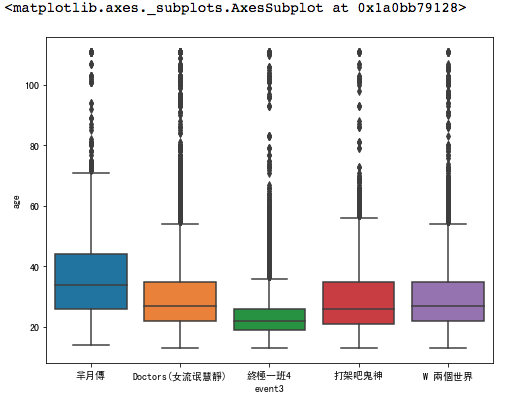情境四: 老闆說: 我想了解整個去年的產品價格趨勢

Ex:

plt.plot(stock[['High','Min']])
plt.legend(["High","Min"], loc=0)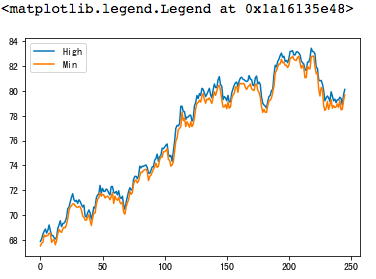Ex:

stock[['Close','High','Min']].plot(subplots = True)
plt.show()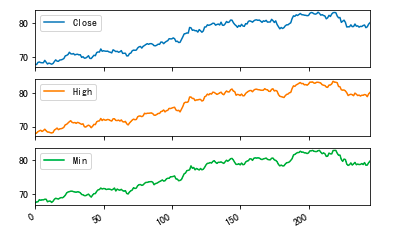情境五: 老闆： 我想知道不同參數之間的Correlation

Ex:

sb.pairplot(mtcars,  diag_kind="kde")

![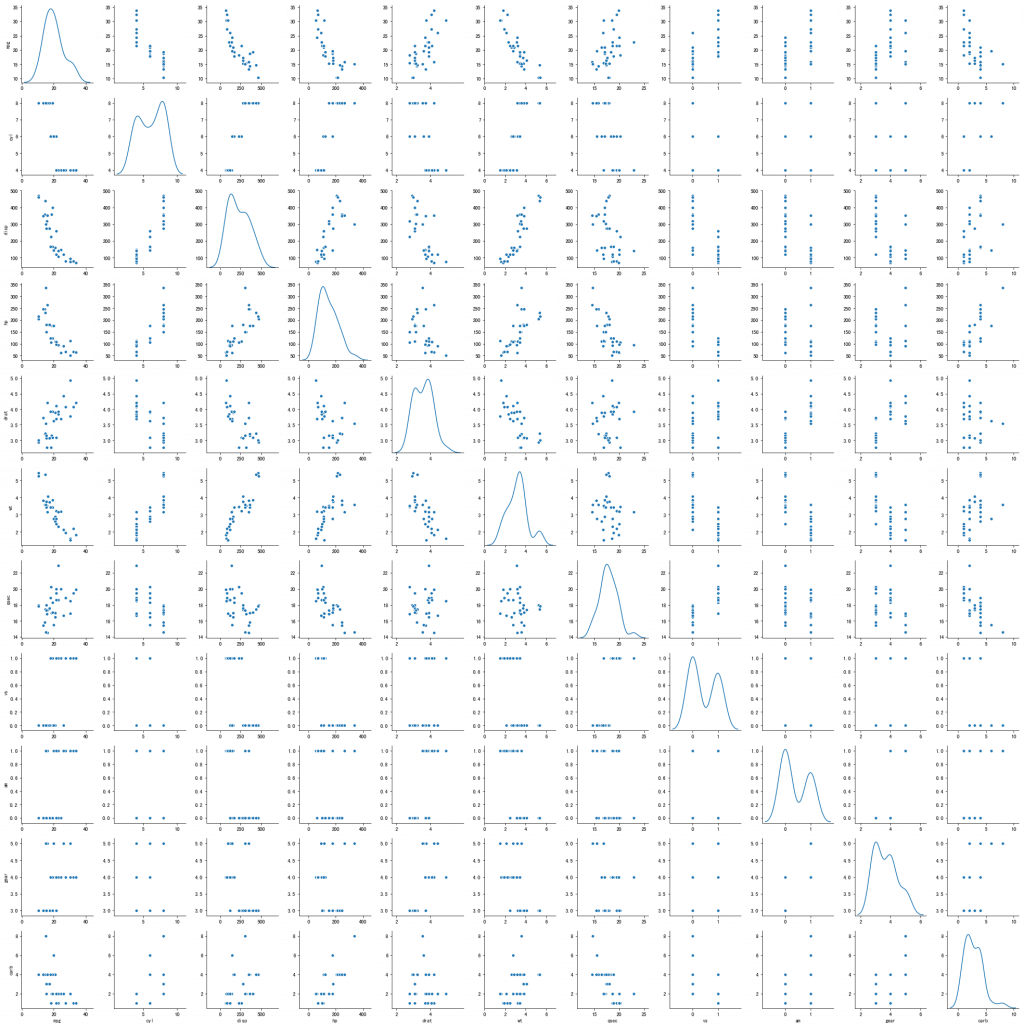corrmat = mtcars.corr()
f, ax = plt.subplots(figsize=(12, 9))
sb.heatmap(corrmat, annot=True)